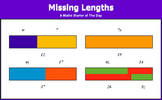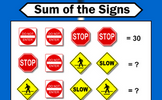# Understand and use algebraic notation

### Term 1 starting in week 3 :: Estimated time: 2 weeks

• Given a numerical input, find the output of a single function machine
• Use inverse operations to find the input given the output
• Use diagrams and letters to generalise number operations
• Use diagrams and letters with single function machines
• Find the function machine given a simple expression
• Substitute values into single operation expressions
• Find numerical inputs and outputs for a series of two function machines
• Use diagrams and letters with a series of two function machines
• Find the function machines given a two-step expression
• Substitute values into two-step expressions
• Generate sequences given an algebraic rule
• Represent one- and two-step functions graphically

This page should remember your ticks from one visit to the next for a period of time. It does this by using Local Storage so the information is saved only on the computer you are working on right now.

## Lesson Starters

Here are some suggestions for whole-class, projectable resources which can be used at the beginnings of each lesson in this block.

### 1st Lesson#### 5.5 Times Table

Write out the 5.5 times table as far as possible.

### 2nd Lesson#### Connecting Rules

Give 20 rules connecting x and y given their values.

### 3rd Lesson#### PYA

You have four minutes to write down as many equations as you can involving the given letters.

### 4th Lesson#### Missing Lengths

Introduce linear equations by solving these problems about lengths.

### 5th Lesson#### Sum of the Signs

Each traffic sign stands for a number. Some of the sums of rows and columns are shown. What numbers might the signs stand for?

### 6th Lesson#### Sea Shells

A question which can be best answered by using algebra.

Some of the Starters above are to reinforce concepts learnt, others are to introduce new ideas while others are on unrelated topics designed for retrieval practice or and opportunity to develop problem-solving skills.

White Rose ResourcesEnd of block assessments provide a quick progress check at the end of each block of learning to make sure students have understood the content covered. This Scheme of Learning was produced by White Rose Maths and is used here with permission granted on 30th June 2021.For All: